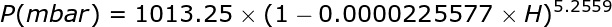# ALTITUDE-PRESSURE CALCULATOR

## CALCULATE ATMOSPHERIC PRESSURE BASED ON ALTITUDE OVER THE SEA LEVEL

The equation used in this calculator is as follows:Pressure in milibar (or hPa) and temperature in and temperature in degrees Celsius (ºC)

It provides the relationship between atmospheric pressure and altitude according to the International Standard Atmosphere (ISA). These values are used as a reference for other calculations. Actual barometric pressure values vary not only with altitude, but also vary with geographic location and weather conditions. At sea level a barometric pressure of 1013.25 mbar is considered as reference, as the altitude increases the pressure decreases.
 Altitud: m Atmospheric Pressure: mmHg bar mbar HPa psi atm mH2O inHg Pa (Pascal)

## CALCULATE ALTITUDE BASED ON ATMOSPHERIC PRESSURE

 Atmospheric pressure: mmHg bar mbar ) HPa psi atm mH2O inHg Pa Altitude: m

Other calculators: